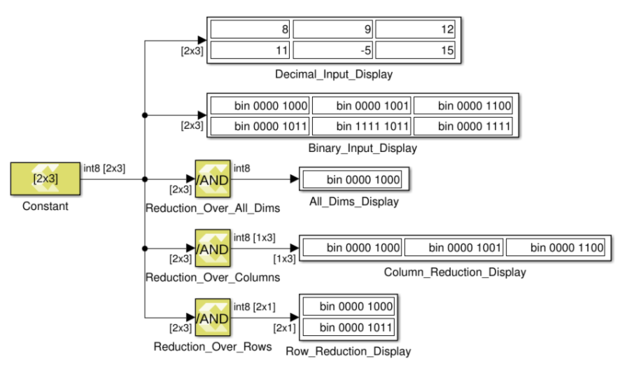# Reduction AND - 2022.1 English

## Vitis Model Composer User Guide (UG1483)

Document ID
UG1483
Release Date
2022-05-26
Version
2022.1 English

Compute bitwise AND of the elements of the input over all dimensions or over a specified dimension

## Library

Logic and Bit Operations## Description

The Reduction AND block has one input signal and one output signal. It computes the bitwise AND of the elements of the input signal over all of the dimensions or over a specified dimension. The data type of the output signal is the same as that of the input signal. The dimension of the output signals depends on whether the reduction takes place over all dimensions or over a specified dimension.

Reduce over all dimensions
The output is a scalar and it is the bitwise AND of the elements of the input signal.
Reduce over dimension 1
The output is a row vector (2-D) with as many elements as the number of columns of the input. Each element in the output is the bitwise AND reduction of the elements of the corresponding column of the input.
Reduce over dimension 2
The output is a column vector (2-D) containing as many elements as the number of rows of the input. Each element in the output is the bitwise AND reduction of the elements of the corresponding row of the input.

In the example below a 2x3 input signal of type int8 feeds into three different configurations of the Reduction AND block.

Figure 1. Reduction AND Block## Data Type Support

• The input signal can be of any data type except for floating point types.
• The input signal must be real.
• The input signal must be a matrix if reduction is along dimension 2.
• The input signal can be a matrix, vector or scalar if reduction is along all dimensions or along dimension 1.

## Parameters

Reduce over

This parameter specifies whether reduction takes place over all dimensions or over a specified dimension. If reduction is specified over all dimensions, the output signal is a scalar.

Following are the settings for the Reduce over parameter.

Setting Description
All dimensions The Reduce AND operator will be applied to all elements, producing a scalar output.
Specified dimension The Reduce AND operator will be applied along the specified dimension, producing a vector output along the opposite dimension. When Dimension 1 is specified, the Reduction AND is applied along columns, producing a row vector as output. When Dimension 2 is specified, the Reduction AND is applied along rows, producing a column vector as output.
Note: The dimension specified will be the one that gets reduced to size 1. For example, a 2-D M x N input matrix specifying Dimension 1 (number of rows M) will result in a 1 x N row vector.

Dimension

If the Reduce over parameter is set to Specified dimension, the Dimension parameter specifies over which dimension reduction takes place.

• If the input signal has dimensions M x N and the reduction Dimension is 1, the output has dimensions 1 x N.
• If the input signal has dimensions M x N and the reduction Dimension is 2, the output has dimensions M x 1.
• If the input signal is scalar or 1 x 1, the output dimension is 1 x 1.

Following are the settings for the Dimension parameter.

Table 1. Dimension Parameter
Setting Description
1 Reduce over row dimension.
2 Reduce over column dimension.
Note: If the reduce Dimension is specified to be 2 the input signal must be two-dimensional.